Continued from main description blog of Ultimate Trade Copier: https://www.mql5.com/en/blogs/post/744859

### Slave Settings - Inputs Block #4 - Indicator FiltersSee Blog post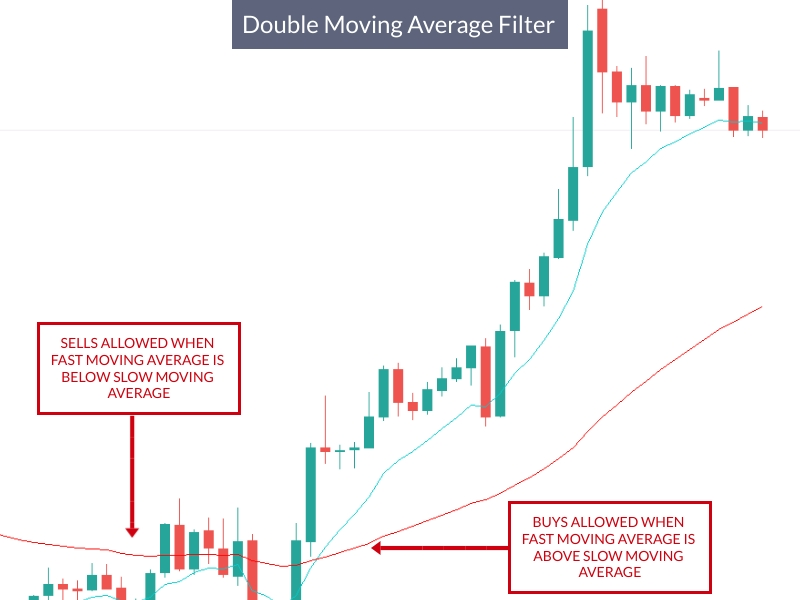• MA Filter Buy Side  — if true, slave will accept buy trades from master only if fast moving average is ABOVE the slow moving average. Default=false.

For instance, maybe you want to copy buy trades when the 5-period SMA is above the 50-period SMA, so you would indicate MA Filter Buy Side=true and configure MA Filter Time Frame = H1, MA Fast Period=5 and MA Slow Period=50 and MA Filter Moving Average Method=SMA.

• MA Filter Sell Side  — if true, slave will accept sell trades from master only if fast moving average is BELOW the slow moving average. Default=false.

• MA Filter Buy Side Reverse     if true, slave will accept buy trades from master only if fast moving average is BELOW the slow moving average. Default=false.

• MA Filter Sell Side Reverse    if true, slave will accept sell trades from master only if fast moving average is ABOVE the slow moving average. Default=false.

• MA Filter Time Frame    indicate the MA Time Frame of the two moving averages: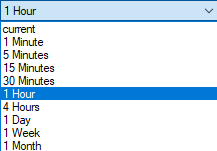• MA Filter Fast Periods  — fast MA indicator period

• MA Filter Slow Periods  — slow MA indicator period

• MA Filter Moving Average Method - indicator method.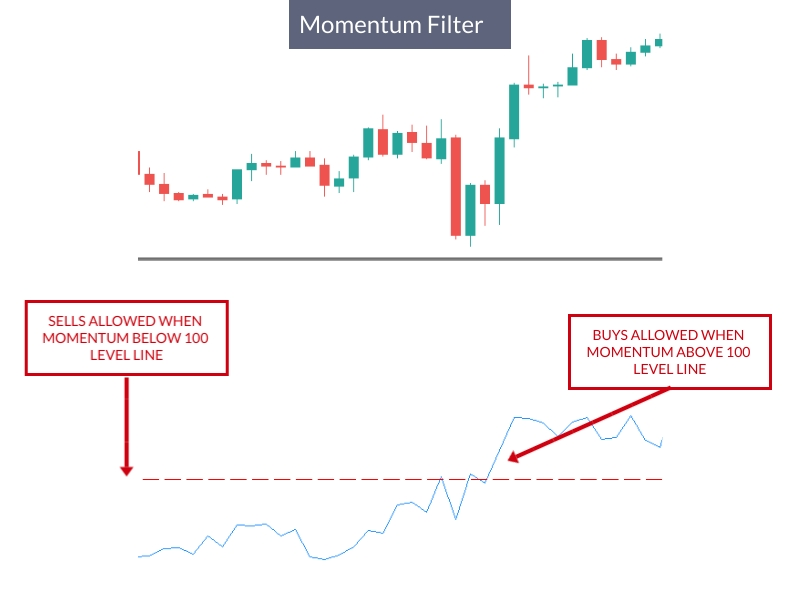• Momentum Filter Buy Side  — if true, slave will accept buy trades from master only if momentum value is ABOVE the momentum level

For instance, maybe you want to take buy trades when the H1 momentum (20) is greater than level=100.2, so you would indicate Momentum Filter Buy Side=true and configure Momentum Time Frame = H1, Momentum Filter Period=20, Momentum Factor=0.2 (level starts at 100, so factor of 0.2, on buy side, adds 0.2 to the level: 100+0.2=100.2)

• Momentum Filter Sell Side  — if true, slave will accept sell trades from master only if momentum value is BELOW the momentum level

For instance, maybe you want to take buy trades when the H1 momentum (20) is below than level=99.8, so you would indicate Momentum Filter Sell Side=true and configure Momentum Time Frame = H1, Momentum Filter Period=20, Momentum Factor=0.2 (level starts at 100, so factor of 0.2, on sell side, subtracts 0.2 from the level: 100-0.2=98.8)

• Momentum Filter Buy Side Reverse  — if true, slave will accept buy trades from master only if momentum value is BELOW the momentum level

• Momentum Filter Sell Side Reverse  — if true, slave will accept sell trades from master only if momentum value is ABOVE the momentum level

• Momentum Filter Time Frame    indicate the Momentum Time Frame:• Momentum Filter Periods    indicate the Momentum Period. Default is 45.

• Momentum Filter Factor   indicate the Momentum Factor to add/substract to level of 100.  Default is 0.2.

•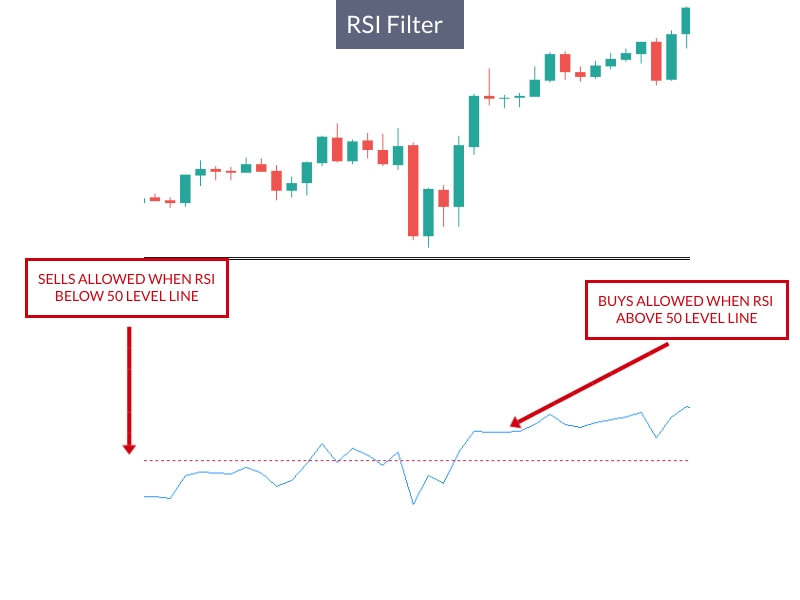• Rsi Filter Buy Side  — if true, slave will accept buy trades from master only if RSI value is ABOVE the RSI level

For instance, maybe you want to take buy trades when the H1 RSI (20) is greater than level=55, so you would indicate RSI Filter Buy Side=true and configure RSI Filter Time Frame = H1, RSI Filter Period=20, and RSI Filter Level=5 (level starts at 50, so Filter Level=5, on buy side, adds 5 to the level: 50+5=55)

• Rsi Filter Sell Side  — if true, slave will accept sell trades from master only if RSI value is BELOW the RSI level

For instance, maybe you want to take sell trades when the H1 RSI (20) is below the level of 45, so you would indicate RSI Filter Sell Side=true and configure RSI Filter Time Frame = H1, RSI Filter Period=20, and RSI Filter Level=5 (level starts at 50, so Filter Level=5, on sell side, subtracts 5 from the level: 50-5=45)

• Rsi Filter Buy Side Reverse  — if true, slave will accept buy trades from master only if RSI value is BELOW the RSI level

• Rsi Filter Sell Side Reverse  — if true, slave will accept sell trades from master only if RSI value is ABOVE the RSI level

• Rsi Filter Time Frame   indicate the Momentum Time Frame:• Rsi Filter Periods  — RSI period. Default is 35.

• Rsi Filter Level  — RSI level 50 (0), and any number is added (subtracted) to 50 on buy (sell) trades.

RSI Filter Level=5 means that you will buy when RSI is above 55 and sell when RSI is below 45.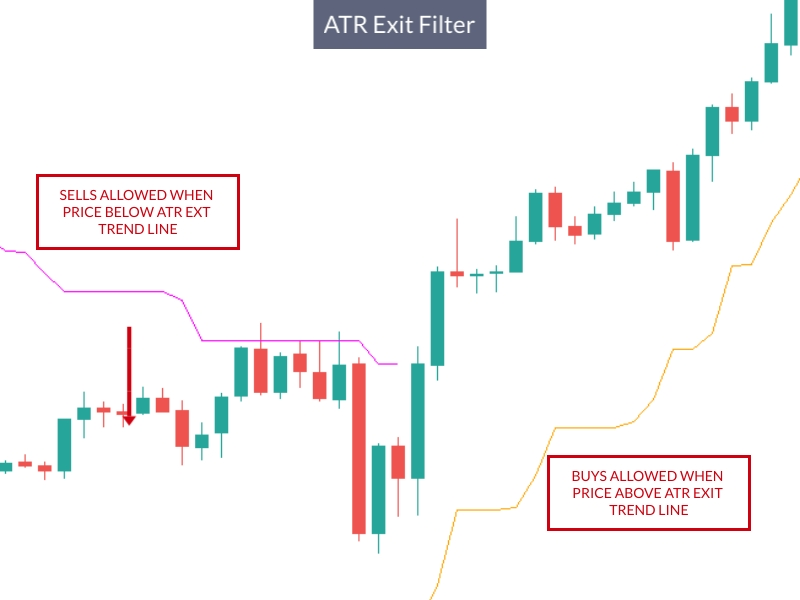• AtrExit Filter Buy Side  — if true, slave will accept buy trades from master only if ATRExit Trend is UP

• AtrExit Filter Sell Side  — if true, slave will accept sell trades from master only if ATRExit Trend is DOWN

• AtrExit Filter Buy Side Reverse  — if true, slave will accept buy trades from master only if ATRExit Trend is DOWN

• AtrExit Filter Sell Side Reverse  — if true, slave will accept buy trades from master only if ATRExit Trend is UP

• AtrExit Filter Time Frame   indicate the ATRExit Time Frame:• Atr Period  ATR Period

• Atr Factor  — ATR Factor